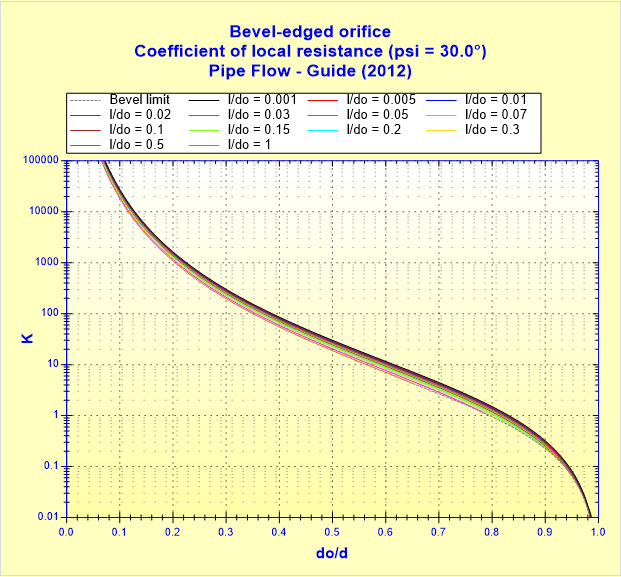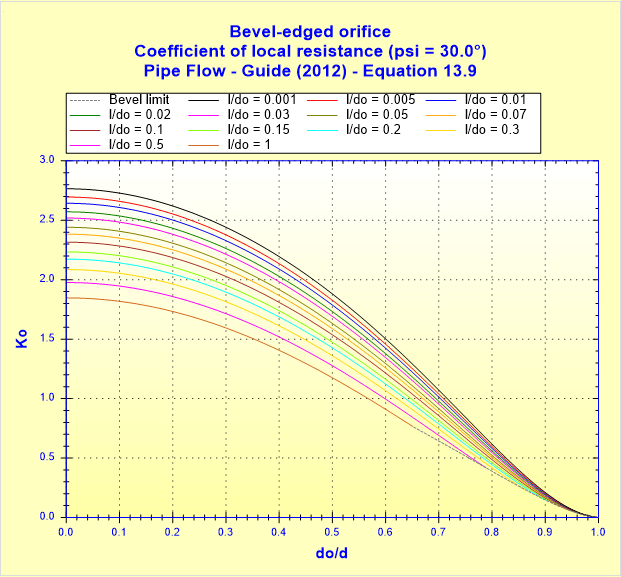# Screenshots## Thick-edged orifice (with transition) – Coefficient of local resistance (t_do _ 1.0) – Pipe Flow – Guide (2012) – Equation 13.16## Thick-edged orifice (with transition) – Coefficient of local resistance (fo _ 0.02) (t_do _ 5.0) – Pipe Flow – Guide (2012) – Equation 13.17## Sharp-edged orifice (with transition) – Coefficient of local resistance – Pipe Flow – Guide (2012) – Equation 13.5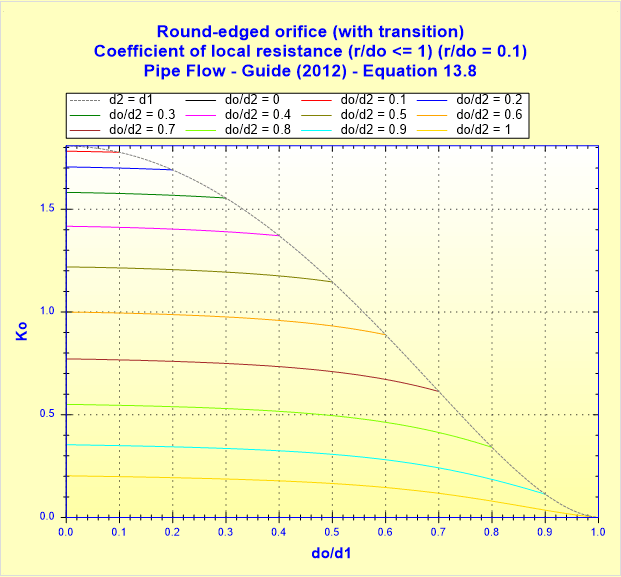## Round-edged orifice (with transition) – Coefficient of local resistance (r_do __ 1) (r_do _ 0.1) – Pipe Flow – Guide (2012) – Equation 13.8## Round-edged orifice (with transition) – Coefficient of local resistance (r_do __ 1) (do_d2 _ 0.5) – Pipe Flow – Guide (2012)## Round-edged orifice (with transition) – Coefficient of local resistance (r_do __ 1) (do_d2 _ 0.5) – Pipe Flow – Guide (2012) – Equation 13.8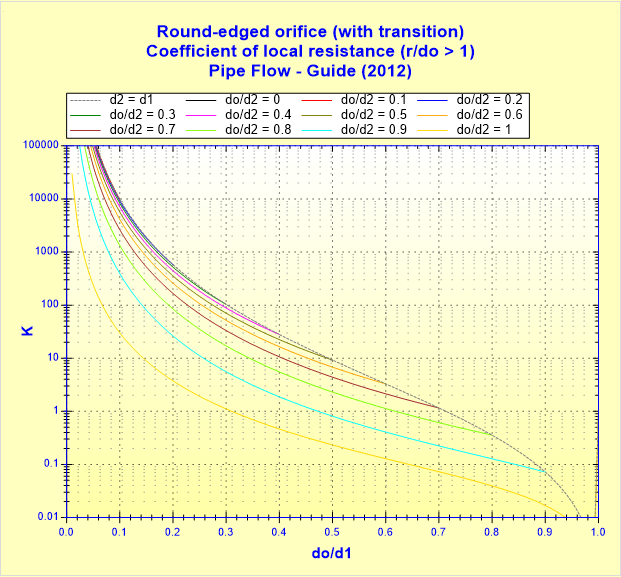## Round-edged orifice (with transition) – Coefficient of local resistance (r_do _ 1) – Pipe Flow – Guide (2012)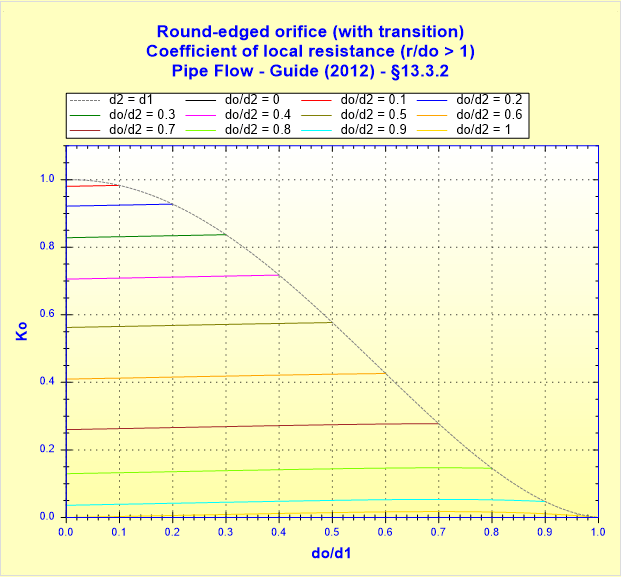## Round-edged orifice (with transition) – Coefficient of local resistance (r_do _ 1) – Pipe Flow – Guide (2012) – §13.3.2## Round-edged orifice (r_do __ 1) – Coefficient of local resistance (K) – Pipe Flow – Guide (2012)## Round-edged orifice (r_do __ 1) – Coefficient of local resistance – Pipe Flow – Guide (2012) – Equation 13.6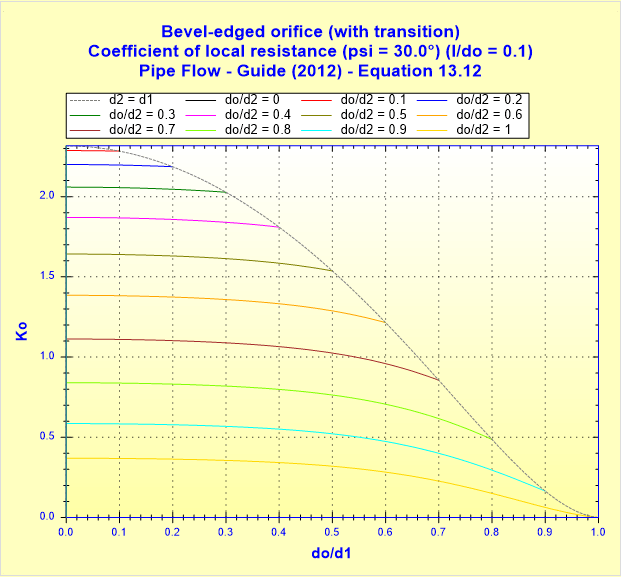## Bevel-edged orifice (with transition) – Coefficient of local resistance (psi _ 30.0°) (l_do _ 0.1) – Pipe Flow – Guide (2012) – Equation 13.12## Bevel-edged orifice (with transition) – Coefficient of local resistance (psi _ 30.0°) (do_d2 _ 0.5) – Pipe Flow – Guide (2012)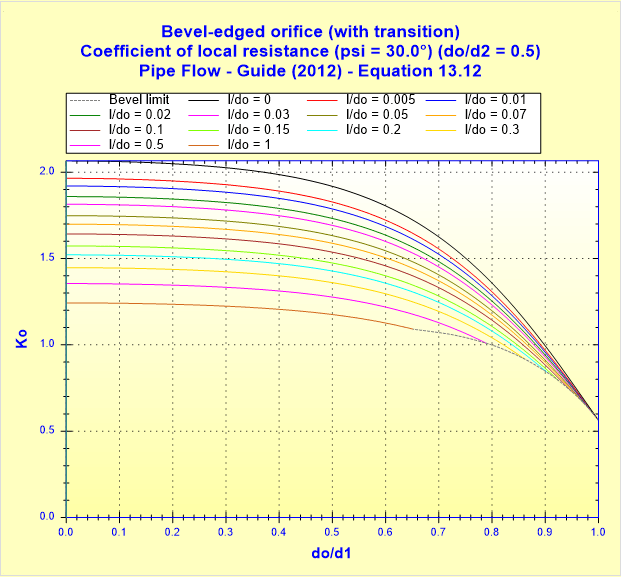## Bevel-edged orifice (with transition) – Coefficient of local resistance (psi _ 30.0°) (do_d2 _ 0.5) – Pipe Flow – Guide (2012) – Equation 13.12## Bevel-edged orifice – Jet velocity ratio (psi _ 75.0°) – Pipe Flow – Guide (2012) – Equation 13.10## Bevel-edged orifice – Jet velocity ratio (psi _ 60.0°) – Pipe Flow – Guide (2012) – Equation 13.10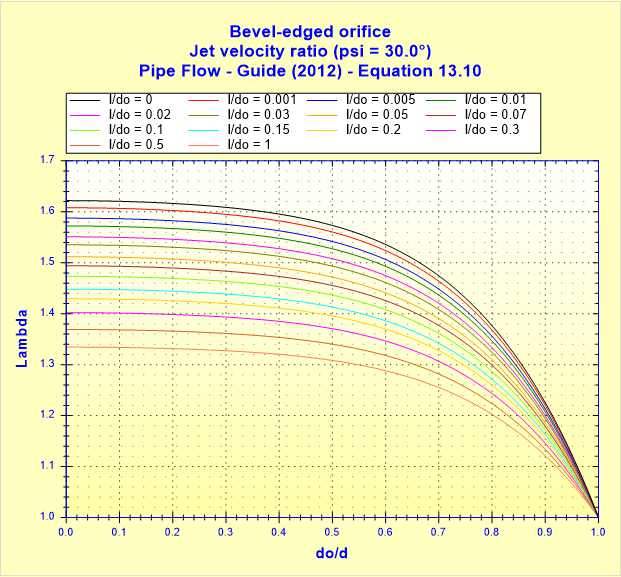## Bevel-edged orifice – Jet velocity ratio (psi _ 30.0°) – Pipe Flow – Guide (2012) – Equation 13.10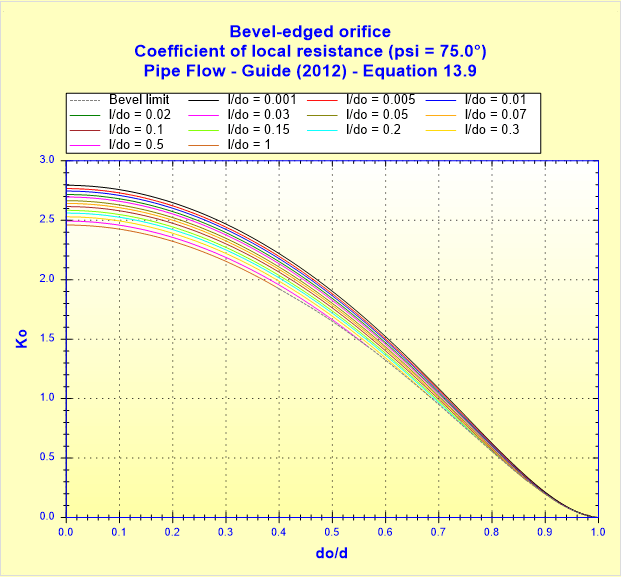## Bevel-edged orifice – Coefficient of local resistance (psi _ 75.0°) – Pipe Flow – Guide (2012) – Equation 13.9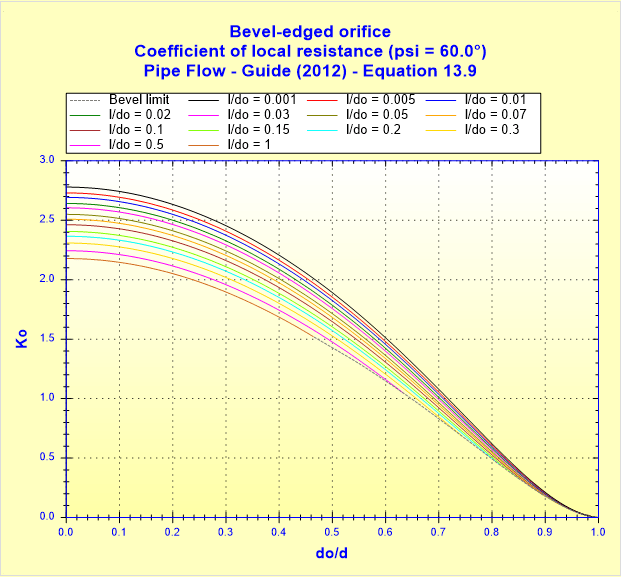## Bevel-edged orifice – Coefficient of local resistance (psi _ 60.0°) – Pipe Flow – Guide (2012) – Equation 13.9